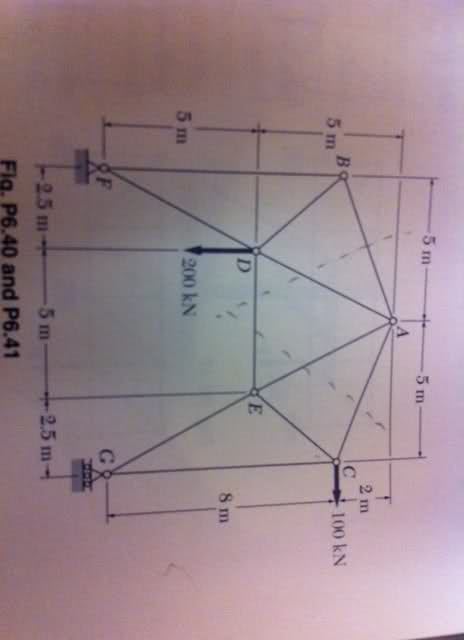# Static 2d body, force in member unknown

• togo

## Homework StatementFind stress or force in member AE

## Homework Equations

torque = force x distance

## The Attempt at a Solution

Solution out of book is 142 kN

Attempts:
Mb = -(200kN)(2.5m) + (.895AE)(5) + 510
= 111 Kn

Mc = -(3m)(170kN) + (.895AE)(5)
= 114 kN

Md = (-80)(2.5) - (100kN)(7.5m) + (.895AE)(2.5)
= 424.60 kN

Solving for force at F and G, Fy = 80 kN and Gy = 130 kN

where did I go wrong?

There are still reaction forces at F and G that you havn't took into consideration.

For B and C the forces at G and F are directly in line therefore they cancel out. In my calculation for D, I did take F into account

Any ideas